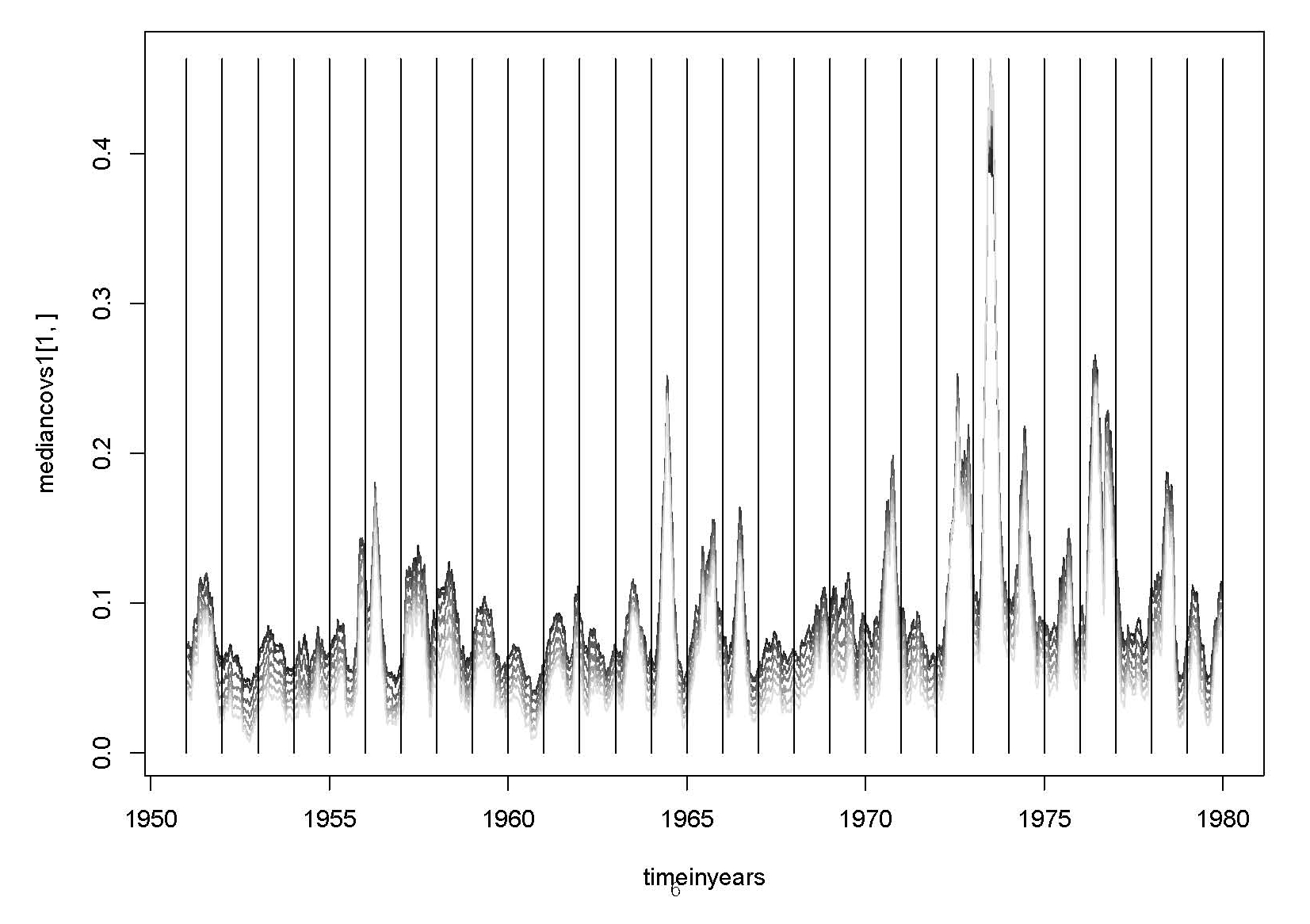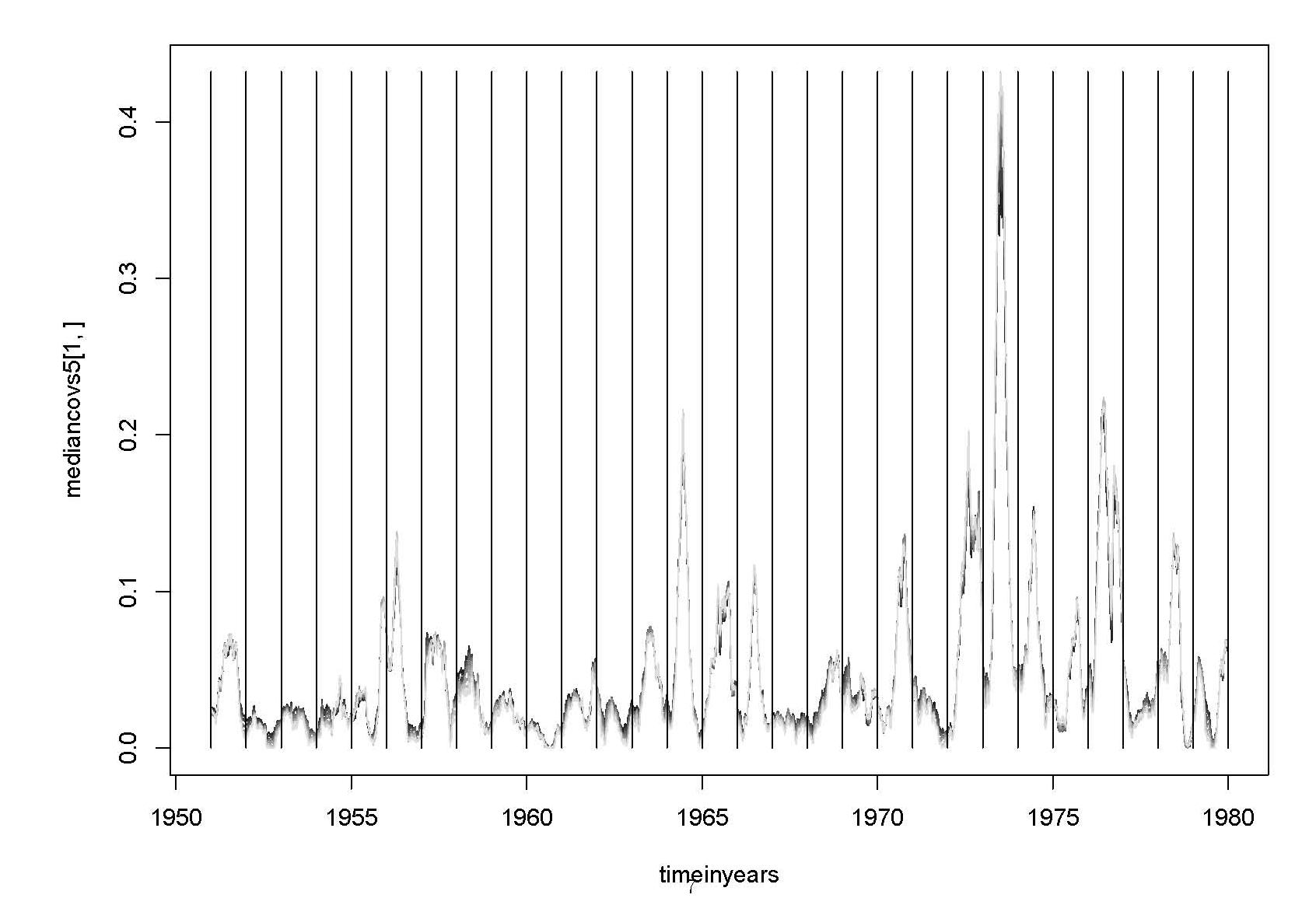# The Azimuth Project Blog - El Nino project (part 9)

This is a blog article in progress, written by John Baez. To see discussions of the article as it was being written, visit the Azimuth Forum.

If you want to write your own article, please read the directions on How to blog.

So far in this series we’ve examined two papers that use very similar methods to construct ‘climate networks’. These papers compute correlations between temperatures at various points on a grid, and then do further computations with these to define a number called a ‘link strength’ between each pair of points. But what if we used covariances instead of correlations?

I’ll explain the difference in a minute, but covariances are simpler, and in the one case Graham Jones has looked at so far, they seem to work just as well. So, they’re worth considering.

Correlations vs covariances in link strength

The graph shows a variant of the Ludescher et al algorithm. The original algorithm using correlations is in black. A variant using covariances is shown in red.

You can get the code on GitHub.

### The Pacific in 1951

The images below show local correlations and covariances of temperatures over the Pacific. Correlations are on the left and covariances on the right. They are calculated over the year of 1951. The correlations are shown on a scale where black is zero, white is 1. All of the values were positive; the smallest correlation was 0.26. The cube roots of the covariances are shown on a scale where black is zero and white is the maximum value. A linear mapping of values just shows a few pale pixels near the corners, with the rest black. Summary values for a set of covariances:

    Min.  1st Qu.   Median     Mean  3rd Qu.     Max.
0.09237  0.22520  0.40570  1.28000  1.11200 22.77000 

### More covariances

The PDF file Covariances near equator shows covariances between different places near the equator in the Pacific and at two different time delays. Most of the details are in the PDF, but some things are not:

• the first graph shows a 1-day delay and the second a 5-day delay.

• the graphs show the median of the covariances over the region

• black means zero geographical displacement, and paler greys show displacements increasing by 2.5 degrees.

The idea is to plot, for each 5 days from 1951 through 1979, for a region straddling the equator, and for 0 to 7 eastwards steps of 2.5 degrees, the covariances of the temperature over six months (183 days). Here are the results:### Covariance maps 1951-1979

The image shows one map of the Pacific for each quarter for the years 1951 through 1979. On the right, the NINO3.4 index is shown for the year.

The area is that used by Ludescher et al. The El Niño basin as defined by these authors is the black region along the Equator towards the East, plus two pixels below. For every other pixel $i$, the sum $\mathrm{TC}(i)$ of the covariances between $i$ and the 14 pixels in the basin is shown. The covariances are calculated over the previous year. The absolute values are ‘squashed’ before conversion to colors. Negative values of $\mathrm{TC}(i)$ are red, positive values green, paler meaning bigger in absolute value. Very big values are shown by bright red and green.

### More detail

The climatological seasonal cycle (mean over years for each grid point, each day-in-year) is subtracted. The data is spatially subsampled into 7.5 by 7.5 degree squares. There are 9 by 23 such squares. The covariances are calculated for a day in the middle of each quarter. The covariances are calculated over a period of 365 days. There is no time delay between the periods. The $\mathrm{TC}(i)$ values are squashed down to values

$\mathrm{sign}(TC(i)) \sqrt(|TC(i)|)$

before conversion to colors. The range -3,3 is mapped to dull shades; below -3 is bright red, above 3 bright green.

The El Niño index is from http://www.cpc.ncep.noaa.gov/products/analysis_monitoring/ensostuff/detrend.nino34.ascii.txt.

To see how this image was made: R code to make covariance maps 1951-1979

### Studying the dependence of covariance on distance

The graphs below are based on David Tanzer’s numbers in this thread in the forum. The top three graphs show the square roots of the absolute values of the covariances. The bottom three are similar, but the values are scaled so that the covariance for the smallest distance is always 1.

Here is the code that generated the underlying data (in csv form): covariance-by-distance.R.

### Time-delayed correlations and covariances at different places and times

Each of the following images show time-delayed cross-correlations and cross-covariances for one grid point versus others in the Pacific. The graphs are arranged geographically, with Australia bottom left. Adjacent graphs represent points 15 degrees apart.

Correlations, end 1956 (not El Niño), not in basin:

Correlations, end 1956 (not El Niño), in basin:

Correlations, end 1957 (El Niño), not in basin:

Correlations, end 1957 (El Niño), in basin:

Covariances, end 1957 (El Niño), in basin, same grid point as last:

Standard deviations for individual grid points, end 1957 (El Niño), in basin. (The point (5,13) is not special here.)

You can get the code for the images here on Github.

category: blog